Next: John Phillips - Spectral Up: Operator Algebras / Algèbres Previous: Michael Lamoureux - Crossed

## Alexandru Nica - Some minimization problems for the free analogue of the Fisher information

 ALEXANDRU NICA, Department of Pure Mathematics, University of Waterloo, Waterloo, Ontario  N2L 3G1, Canada Some minimization problems for the free analogue of the Fisher information

We consider the free non-commutative analogue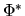, introduced by D. Voiculescu, of the concept of Fisher information for random variables. We determine the minimal possible value of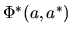, if a is a non-commutative random variable subject to the constraint that the distribution of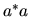is prescribed. More generally, we obtain the minimal possible value of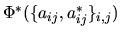, if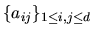is a family of non-commutative random variables such that the distribution of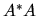is prescribed, where A is the matrix (aij)i,j=1d. The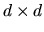-generalization is obtained from the case d=1 via a result of independent interest, concerning the minimal value ofwhen the matrix A = (aij)i,j=1d and its adjoint have a given joint distribution. (A version of this result describes the minimal value of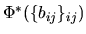when the matrix B = (bij)i,j=1d is selfadjoint and has a given distribution.)

We then show how the minimization results obtained forlead to maximization results concerning the free entropy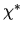, also defined by Voiculescu.

This is joint work with Dimitri Shlyakhtenko and Roland Speicher.Next: John Phillips - Spectral Up: Operator Algebras / Algèbres Previous: Michael Lamoureux - Crossed